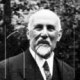# The Unapologetic Mathematician

## The Unit and Counit of an Adjunction

Let’s say we have an adjunction$F\dashv G:\mathcal{C}\rightarrow\mathcal{D}$. That is, functors$F:\mathcal{C}\rightarrow\mathcal{D}$ and$G:\mathcal{D}\rightarrow\mathcal{C}$ and a natural isomorphism$\Phi_{C,D}:\hom_\mathcal{D}(F(C),D)\rightarrow\hom_\mathcal{C}(C,G(D))$.

Last time I drew an analogy between equivalences and adjunctions. In the case of an equivalence, we have natural isomorphisms$\eta:1_\mathcal{C}\rightarrow G\circ F$ and$\epsilon:F\circ G\rightarrow1_\mathcal{D}$. This presentation seems oddly asymmetric, and now we’ll see why by moving these structures to the case of an adjunction.

So let’s set$D=F(C')$ like we did to show that an equivalence is an adjunction. The natural isomorphism is now$\Phi_{C,F(C')}:\hom_\mathcal{D}(F(C),F(C'))\rightarrow\hom_\mathcal{C}(C,G(F(C'))$. Now usually this doesn’t give us much, but there’s one of these hom-sets that we know has a morphism in it: if$C'=C$ then$1_{F(C)}\in\hom_\mathcal{D}(F(C),F(C))$. Then$\Phi_{C,F(C)}(1_{F(C)})$ is an arrow in$\mathcal{C}$ from$C$ to$\left[G\circ F\right](C)$.

We’ll call this arrow$\eta_C$. Doing this for every object$C\in\mathcal{C}$ gives us all the components of a natural transformation$\eta:1_\mathcal{C}\rightarrow G\circ F$. For this, we need to show the naturality condition$G(F(f))\circ\eta_C=\eta_{C'}\circ f$ for each arrow$f:C\rightarrow C'$. This is a straightforward calculation:$G(F(f))\circ\eta_C=G(F(f))\circ\Phi_{C,F(C)}(1_{F(C)})=\Phi_{C,F(C')}(F(f)\circ1_{F(C)})=$$\Phi_{C,F(C')}(1_{F(C')}\circ F(f))=\Phi_{C',F(C')}(1_{F(C')})\circ f=\eta_{C'}\circ f$
using the definition of$\eta_C$ and the naturality of$\Phi$.

This natural isomorphism$\eta$ is called the “unit” of the adjunction$F\dashv G$. Dually we can set$C=G(D)$ and extract an arrow$\eta_D=\Phi_{G(D),D}^{-1}(1_{G(D)})$ for each object$D\in\mathcal{D}$ and assemble them into a natural transformation$\eta:F\circ G\rightarrow1_\mathcal{D}$ called the “counit”. If both of these natural transformations are natural isomorphisms, then we have an equivalence.

For a particular example, let’s look at this in the case of the free-monoid functor$M$ as the left adjoint to the underlying-set monoid$U$. The unit will give an arrow$\eta_S:S\rightarrow U(M(S))$, which here is just the inclusion of the generators (elements of$S$) as elements of the underlying set of the free monoid. The counit, on the other hand, will give an arrow$\epsilon_N:M(U(N))\rightarrow N$. That is, we take all elements of the monoid$N$ and use them as generators of a new free monoid — write out “words” where each “letter” is a whole element of$N$. Then to take such a word and send it to an element of$N$, we just take all the letters and multiply them together as elements of$N$. Since we gave a description of$\Phi$ last time for this case, it’s instructive to sit down and work through the definitions of$\eta_S=\Phi_{S,M(S)}$ and$\epsilon=\Phi_{U(N),N}^{-1}$ to show that they do indeed give these arrows.

July 17, 2007 - Posted by | Category theory

## 11 Comments »

1. And this unit, together with the functor GF and the map GFGF -> GF are precisely the data that the Haskell crowd (including me and sigfpe) are so excited about, calling it “monad” and viewing as the best thing since sliced bread for programming.

This is, IIRC, the historically first neat place that the things were found in. Their use for programming is MUCH later at any rate.Comment by Mikael Johansson | July 17, 2007 | Reply

2. Don’t get too far ahead, there. Before I talk about monads I’ll need to go back and redo monoids.Comment by John Armstrong | July 17, 2007 | Reply

3. I seem to keep on thinking in terms of the unapologetic mathematician anno 2010 or something like that. 😉Comment by Mikael Johansson | July 18, 2007 | Reply

4. Well, after polishing off universals it’s time to show what neat things you can do with monoidal categories. In particular, I’ll deal with internalization, which gives the idea of a “monoid object” in a category. Then a monad on a category is a monoid object in the category of endofunctors on the category.

Incidentally, I’m a little surprised you’re interested in monads for the programming applications rather than for those to homology theory.Comment by John Armstrong | July 18, 2007 | Reply

5. […] on units and counits Last time we took an adjunction and came up with two natural transformations, weakened versions of the […]

Pingback by More on units and counits « The Unapologetic Mathematician | July 18, 2007 | Reply

6. I was just thinking this was looking as if it was heading towards triples (or ‘standard constructions’ or ‘monads’ depending on whom you talk to). As you say, the main place I’ve come across these is in terms of homology theories (especially the work of Barr and Beck), but it doesn’t entirely surprise me to hear that they’re relevant to functional programming in some way – I understand quite a bit of category theory is.Comment by Nicholas Jackson | July 18, 2007 | Reply

7. It’s odd, really. I dropped the computer science half of my major as an undergraduate after I was the one person in a 100+ student class who really loved programming in ML. It made me realize that I was too much a mathematician to really finish off that other program as well.

And then it was years later that I realized that functional programming semantics are all category theory. Even before I knew from categories, I evidently picked up on their elegance.Comment by John Armstrong | July 18, 2007 | Reply

8. […] we can specify an adjunction by its unit and counit. In this case the compatibility in question is a pair of equations of natural transformations: […]

Pingback by Transformations of Adjoints « The Unapologetic Mathematician | July 30, 2007 | Reply

9. […] connected to properties of adjoints. First off, we know that and . This essentially expresses the unit and counit of the adjunction. For the antitone version, let’s show the analogous statement more […]

Pingback by Galois Connections « The Unapologetic Mathematician | May 18, 2009 | Reply

10. Reblogged this on Human Mathematics.Comment by isomorphismes | May 18, 2015 | Reply

11. […] The Unit and Counit of an Adjunction […]Pingback by Le blog du Professeur John Armstrong : « The unapologetic mathematician  | «HENOSOPHIA τοποσοφια μαθεσις υνι√ερσαλις οντοποσοφια | February 7, 2016 | Reply